Next: Surface Elongation Up: Description of Three Dimensional Previous: Surface Curvature

# Absolute Surface Area

The surfaces of man-made objects typically have fixed surface areas, and many natural objects also have surfaces whose areas fall within constrained ranges. Thus surface area is a good constraint on the identity of a surface. Consequently, we would like to estimate the absolute surface area of a segmented surface region, which is possible given the information in the surface image. Estimation applies to the reconstructed surface hypotheses (Chapter 4).

The constraints on the estimation process are:

• The surface region image area is the number of pixels inside the surface region boundaries.
• The image area is related to the surface area of a fronto-parallel planar surface at a given depth by the camera parameters.
• The area of a fronto-parallel planar surface is related to the area of an oriented planar surface by its surface orientation.
• The area of a curved surface is related to the area of the equivalent projected planar surface by the magnitude of its curvature.

The effects of surface curvature are approximately overcome using two correction factors for the principal curvatures, which are calculated in the following manner. In Figure 6.11 part (a), the case of a single dimension is considered. A curved segment of curvaturesubtends an angle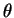. Hence, it has length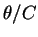. This appears in the image as a straight segment of length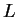. So, the curvature correction factor relating the length of a curved segment to the equivalent chord is: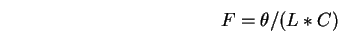where the angleis given by: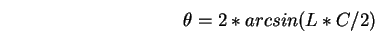Hence, the complete curvature correction factor is (if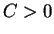):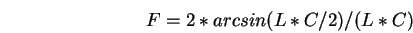else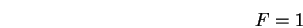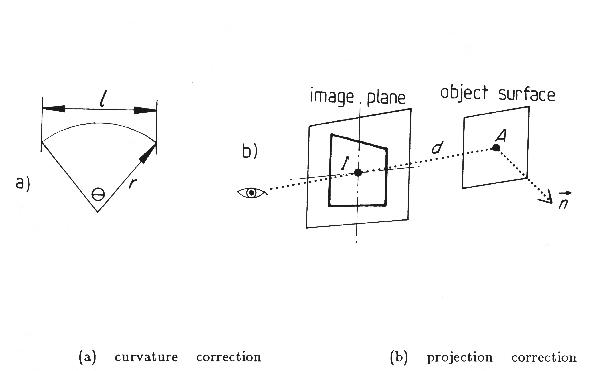Now, referring to Figure 6.11 part (b), the absolute surface area is estimated as follows:

 Let: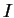be the image area in square pixelsbe the depth to a nominal point in the surface region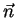be the unit surface normal at the nominal point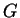be the conversion factor for the number of pixels per centimeter of object length when seen at one centimeter distance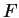,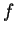be the major and minor principal curvature correction factors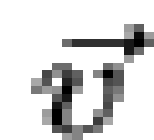be the unit vector from the nominal point to the viewer Then, the slant correction relating projected planar area to true planar area is given by: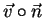and the absolute surface area is estimated by: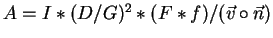Theterm converts one image dimension from pixels to centimeters, theterm accounts for the surface being slanted away from the viewer, and the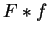term accounts for the surface being curved instead of flat.

In the test image, several unobscured regions from known object surfaces are seen. The absolute surface area for these regions is estimated using the computation described above, and the results are summarized in Table 6.8.

Table 6.8: Summary of Absolute Surface Area Estimation
IMAGE REGION PLANAR OR CURVED ESTIMATED AREA TRUE AREA % ERROR
8 C 1239 1413 12
9 C 1085 1081 0
16 C 392 628 38
26 P 165 201 17
29 C 76 100 24

Note that the estimation error percentage is generally small, given the range of surface sizes. The process is also often accurate, with better results for the larger surface regions and largest errors on small or nearly tangential surfaces. The key sources of error are pixel spatial quantization, which particularly affects small regions, and inaccurate shape data estimation.

From the above discussion, the estimation process is obviously trivial, given the surface image as input. Since the goal of the process is only to acquire a rough estimate, the implemented approach is adequate.Next: Surface Elongation Up: Description of Three Dimensional Previous: Surface Curvature
Bob Fisher 2004-02-26